Next: Scores are inconsistent to Up: Dayhoff Scores and Evolutionary Previous: Dayhoff Scores and Evolutionary

## Monotonicity of scores and distances

Naturally we would assume that a lower PAM distance corresponds to a higher score, i.e. a higher probability that the sequences are related. First we show that this assumption is true when the lengths of the sequences are the same. To verify if smaller PAM distances correspond to larger scores, we have to look at the expected value of the score SD(d) as a function of the PAM distance d. To do this we look at sequences with length n. The expected score SD(d) for each aligned amino acid is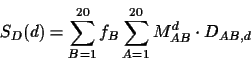where DAB,d is the score of amino acids A and B at PAM distance d and the probabilities are from equation 1. In our case, Dd is a Dayhoff matrix for PAM distance d. If we want the expected score for the whole sequences, we have to multiply this value by n. Calculating SD(d) for each d from 0 to 200, we get the plot in Figure 2.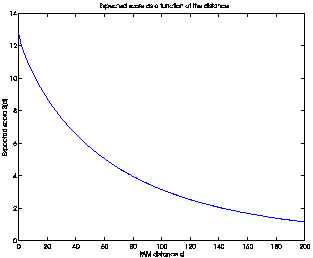The function is monotonic and decreasing, meaning that a larger PAM distance d does correspond to a lower score SD(d). The graph is only a "visual" proof. To prove this, we have to analyze the derivative of SD(d) with respect to d. To compute this derivative, Md can be rewritten as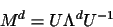where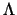is a diagonal matrix containing the eigenvalues of M. Ineach diagonal element is. For any matrix E, SE(d) can be rewritten as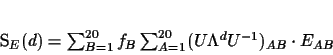(2)

If we multiply everything out we can rewrite SE(d) as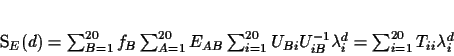(3)

where Tii is the ith diagonal entry in the matrix T = U-1F ET U (F is a diagonal matrix with the frequencies Fii = fi). Its derivative is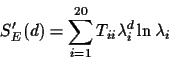and can be computed and verified to be negative for all d in the range 0 to 250, for the Dayhoff matrix in .Next: Scores are inconsistent to Up: Dayhoff Scores and Evolutionary Previous: Dayhoff Scores and Evolutionary
Chantal Korostensky
1999-07-14